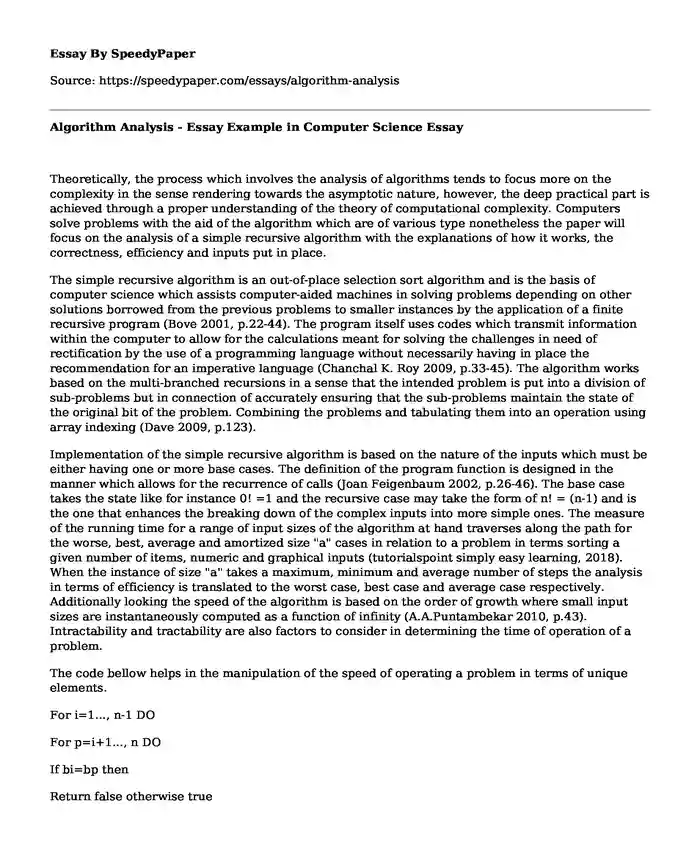# Algorithm Analysis - Essay Example in Computer Science

Published: 2022-06-08Type of paper: Essay Categories: Computer science Pages: 3 Wordcount: 791 words
143 views

Theoretically, the process which involves the analysis of algorithms tends to focus more on the complexity in the sense rendering towards the asymptotic nature, however, the deep practical part is achieved through a proper understanding of the theory of computational complexity. Computers solve problems with the aid of the algorithm which are of various type nonetheless the paper will focus on the analysis of a simple recursive algorithm with the explanations of how it works, the correctness, efficiency and inputs put in place.Is your time best spent reading someone else’s essay? Get a 100% original essay FROM A CERTIFIED WRITER!

The simple recursive algorithm is an out-of-place selection sort algorithm and is the basis of computer science which assists computer-aided machines in solving problems depending on other solutions borrowed from the previous problems to smaller instances by the application of a finite recursive program (Bove 2001, p.22-44). The program itself uses codes which transmit information within the computer to allow for the calculations meant for solving the challenges in need of rectification by the use of a programming language without necessarily having in place the recommendation for an imperative language (Chanchal K. Roy 2009, p.33-45). The algorithm works based on the multi-branched recursions in a sense that the intended problem is put into a division of sub-problems but in connection of accurately ensuring that the sub-problems maintain the state of the original bit of the problem. Combining the problems and tabulating them into an operation using array indexing (Dave 2009, p.123).

Implementation of the simple recursive algorithm is based on the nature of the inputs which must be either having one or more base cases. The definition of the program function is designed in the manner which allows for the recurrence of calls (Joan Feigenbaum 2002, p.26-46). The base case takes the state like for instance 0! =1 and the recursive case may take the form of n! = (n-1) and is the one that enhances the breaking down of the complex inputs into more simple ones. The measure of the running time for a range of input sizes of the algorithm at hand traverses along the path for the worse, best, average and amortized size "a" cases in relation to a problem in terms sorting a given number of items, numeric and graphical inputs (tutorialspoint simply easy learning, 2018). When the instance of size "a" takes a maximum, minimum and average number of steps the analysis in terms of efficiency is translated to the worst case, best case and average case respectively. Additionally looking the speed of the algorithm is based on the order of growth where small input sizes are instantaneously computed as a function of infinity (A.A.Puntambekar 2010, p.43). Intractability and tractability are also factors to consider in determining the time of operation of a problem.

The code bellow helps in the manipulation of the speed of operating a problem in terms of unique elements.

For i=1..., n-1 DO

For p=i+1..., n DO

If bi=bp then

Return false otherwise true

The analysis involving the correctness of algorithm requires proof from the obtained answers. An effective algorithm correctness determined by following the instances of the problem all the way to the end result. The set of the constant point must always be checked to avoid arising errors in manipulating instructions. The design by contract and formal methods are the most important factors to determine whether a program is correct or not. The choice of loop invariants help the computer to relay the information meant to be executed during programming and this was the settlement point on the invariant for the simple recursive algorithm. The algorithm complexity in big "O" is used in the description of the performance operation in terms of complexity of an algorithm and to be specific, it focuses on the scenario involving the worst case. It can further describe the proportion and linear growth with the assistance of loops, for instance, O (N) describes an algorithm in consideration of the size of the input data in terms of performance.

In comparison with the backtracking algorithm, the executions of data are done at a faster speed and inputs of a wider variety of simple recursive algorithm since it has a higher complexity.

References

A.A.Puntambekar. (2010). Design and analysis of algorithms. London: Technical Publications.

Bove, A. (2001). Simple general recursion in type theory. Nordic Journal of Computing, 22-44.

Chanchal K. Roy, J. R. (2009, May 1). Comparison and evaluation of code clone detection techniques and tools: A qualitative approach. computers, pp. 33-45.

Dave, P. H. (2009). Design and Analysis of Algorithms. Chennai: Pearson Education India.

Joan Feigenbaum, S. K. (2002). An Approximate L1-Difference Algorithm for Massive Data Streams. Bankok: Siam publishers.

tutorialspoint simply easy learning. (2018, January 14). DAA: Analysis of algorithms. Retrieved June 1, 2018, from tutorialspoint simplyeasylearning.com: https://www.tutorialspoint.com/design_and_analysis_of_algorithms/analysis_of_algorithms.htm

Algorithm Analysis - Essay Example in Computer Science. (2022, Jun 08). Retrieved from https://speedypaper.com/essays/algorithm-analysis

Request Removal

If you are the original author of this essay and no longer wish to have it published on the SpeedyPaper website, please click below to request its removal:

Liked this essay sample but need an original one?

Hire a professional with VAST experience!